Function Repository Resource:

TextToKaleidoscope

Create a square-symmetric image from a long string of text

Contributed by: Daniel Lichtblau
 ResourceFunction["TextToKaleidoscope"][str] converts the string str into a square image with rotational and reflectional symmetries. ResourceFunction["TextToKaleidoscope"][str,level] converts the string str into a square image of dimensions 2level×2level.

Details and Options

ResourceFunction [ "TextToKaleidoscope" ] uses an encoding of text characters to generate an image.
Characters become five-digit numbers in base 2, with the first two determining where to increment a pixel value (via the "Frequency Chaos Game Representation") and the last three encoding the color channels to be updated.
The dimension exponent must be a whole number in the range 2–10, and the default value is 7.
Setting SymmetrizedFalse gives an image without rotational and reflectional symmetries.
ColorScheme can be set to an integer to seed the random generator, Automatic (so a random seed will be selected automatically) or any of prebuilt schemes "K1", "K2", , "K9".

Examples

Basic Examples

Create an image from a modern English version of Beowulf:

 In:=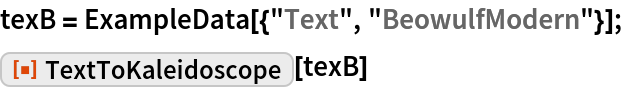Out=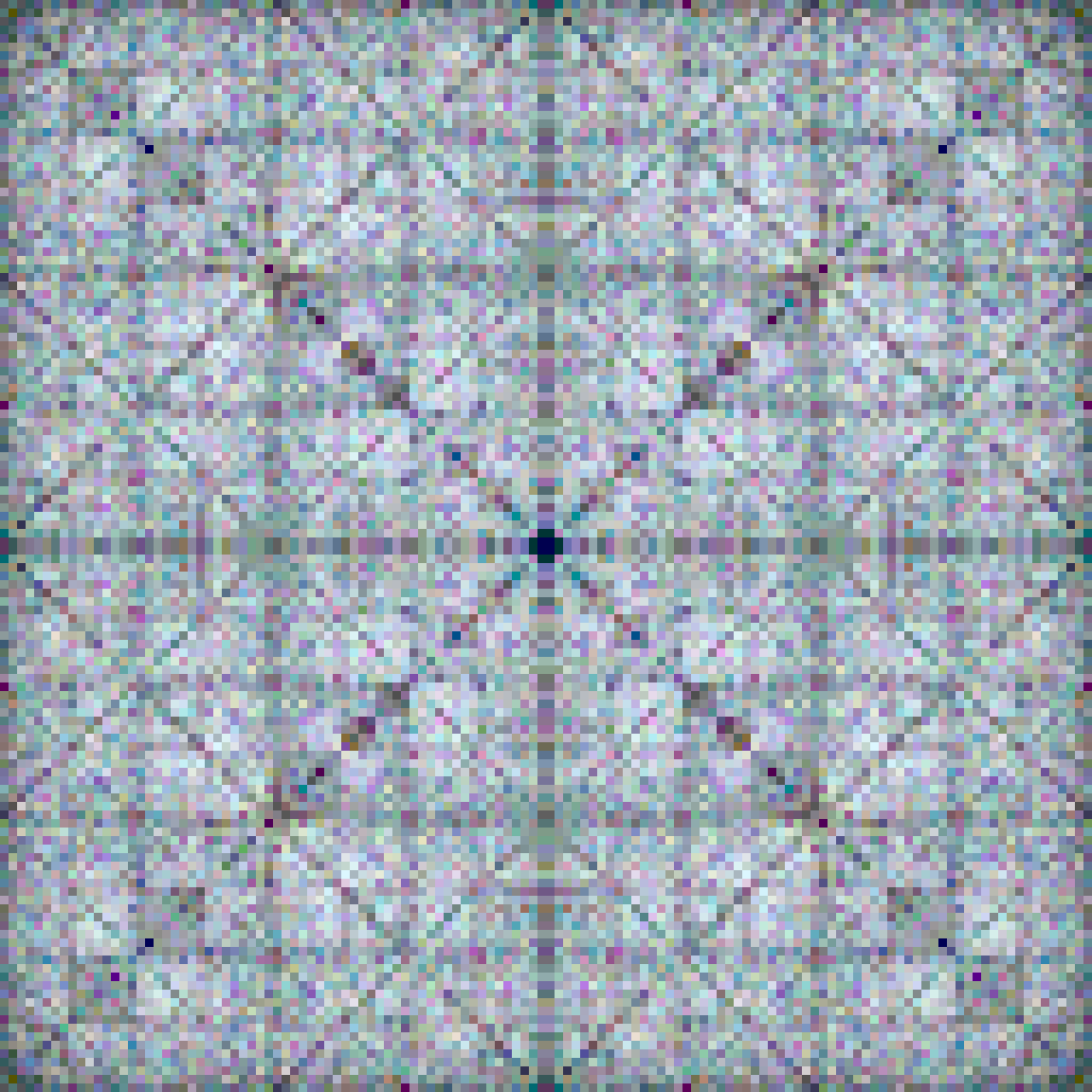Scope

Here are the prebuilt color schemes, as applied to Alice in Wonderland:

 In:=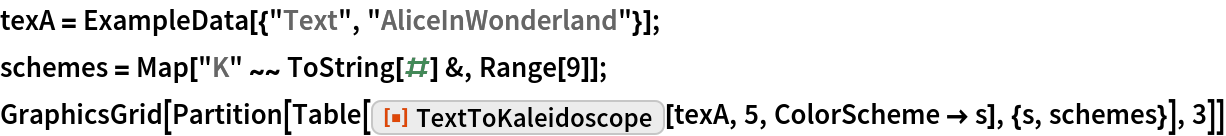Out=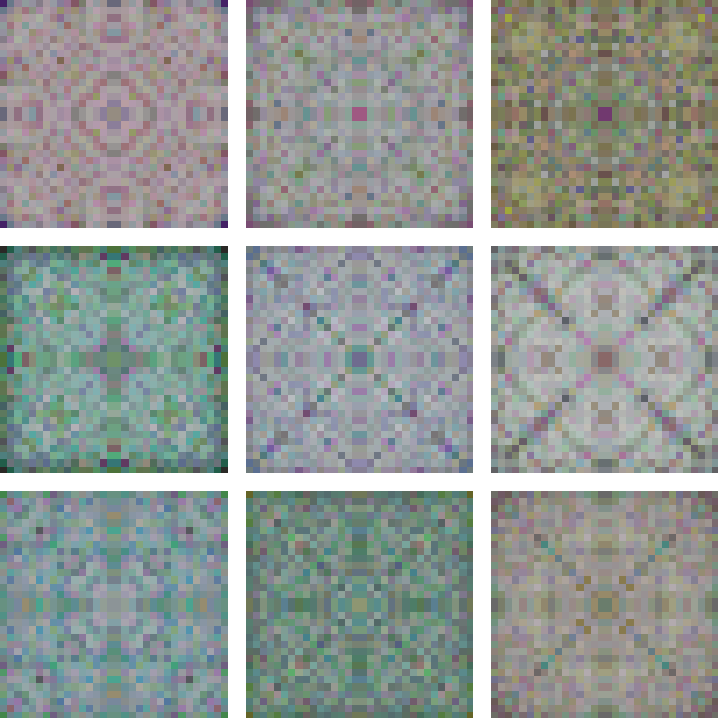A similar but more detailed set of images is shown at pixelation level 9:

 In:=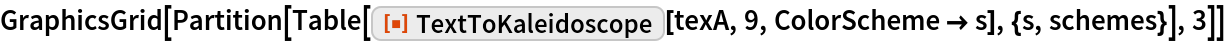Out=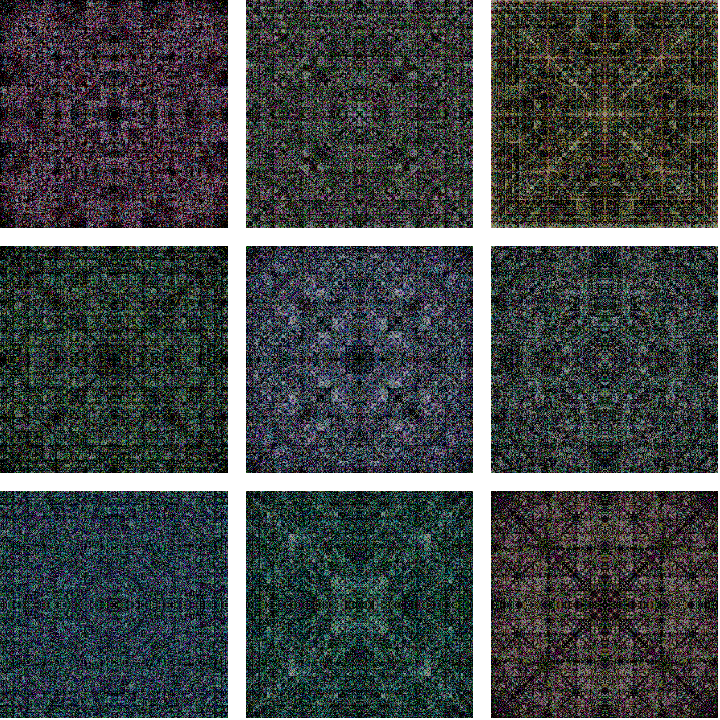Options

Here is an unsymmetrized image computed from Beowulf:

 In:=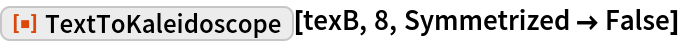Out=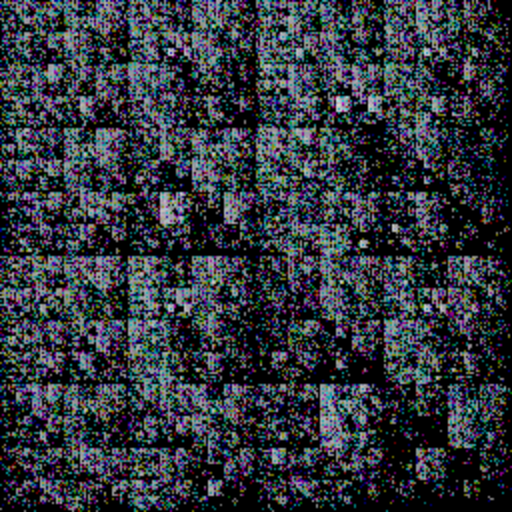TextToKaleidoscope will automatically create a random color scheme if so desired:

 In:=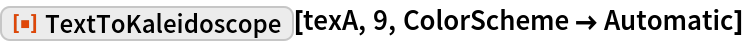Out=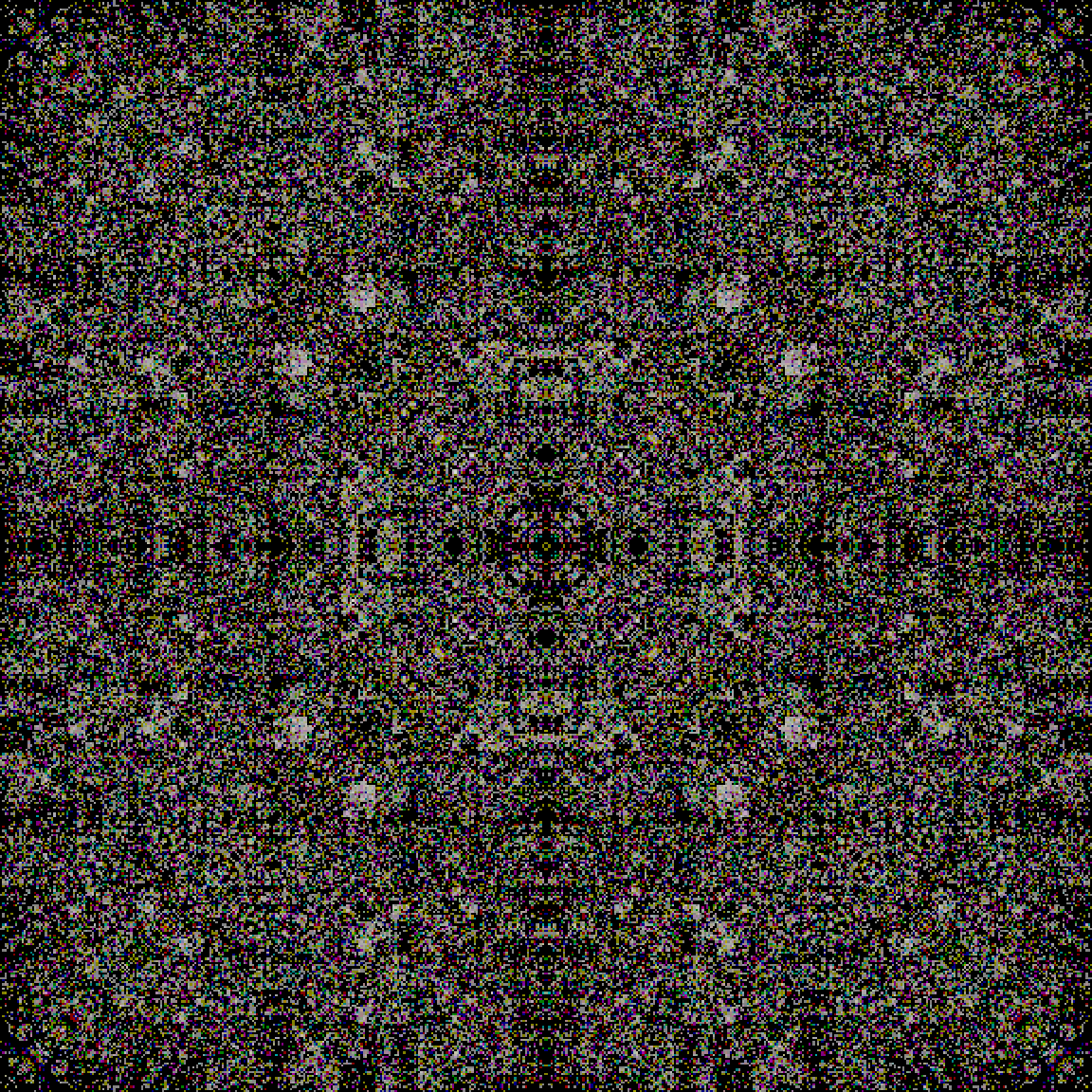TextToKaleidoscope does not change the internal state of the random generators, so repeating the above results in the same image:

 In:=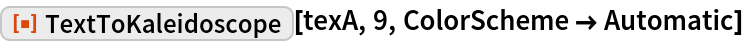Out=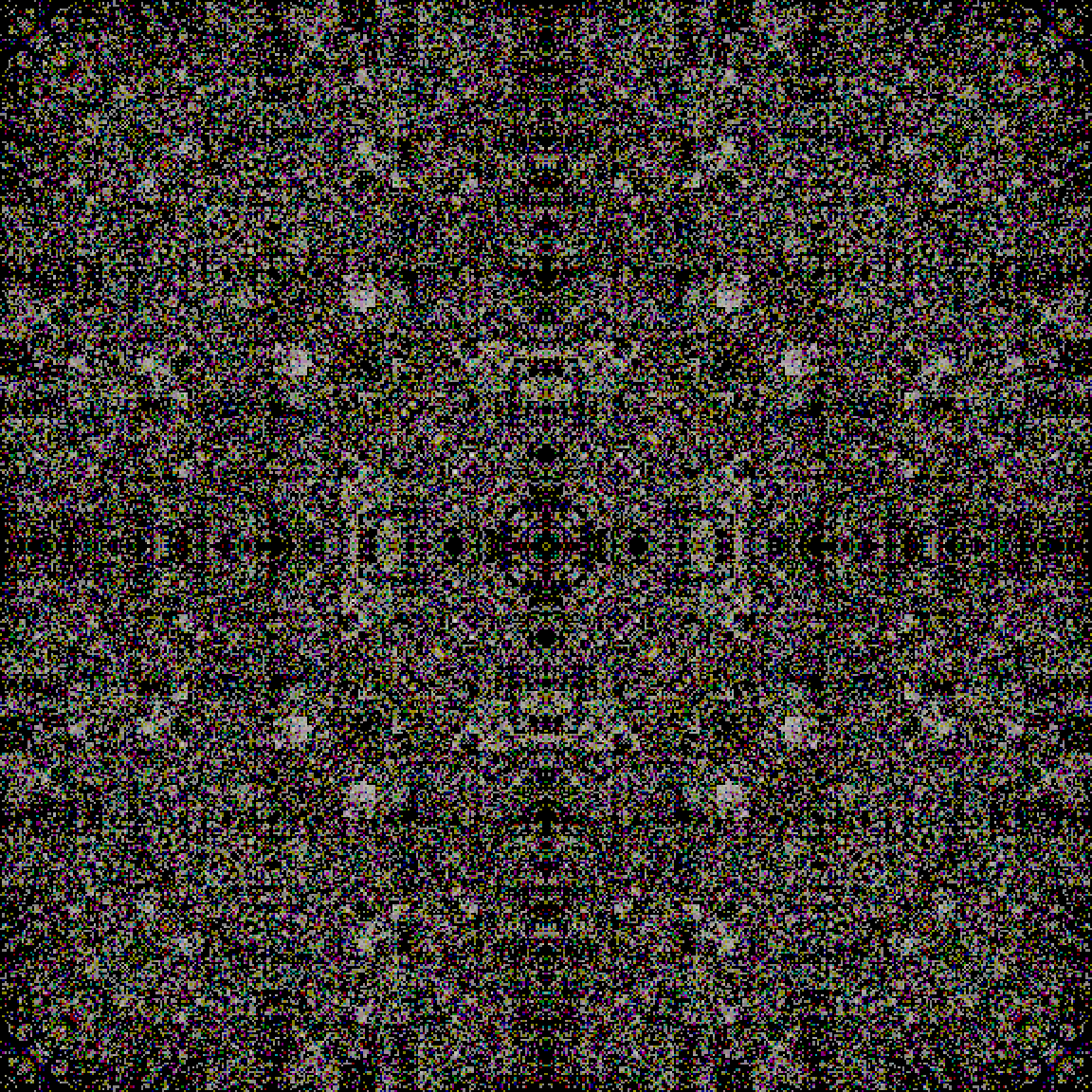Explicitly change the internal random state to get a new randomized color scheme:

 In:=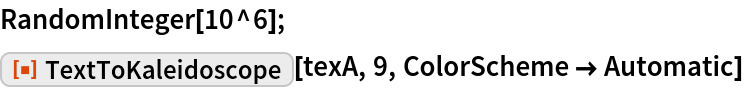Out=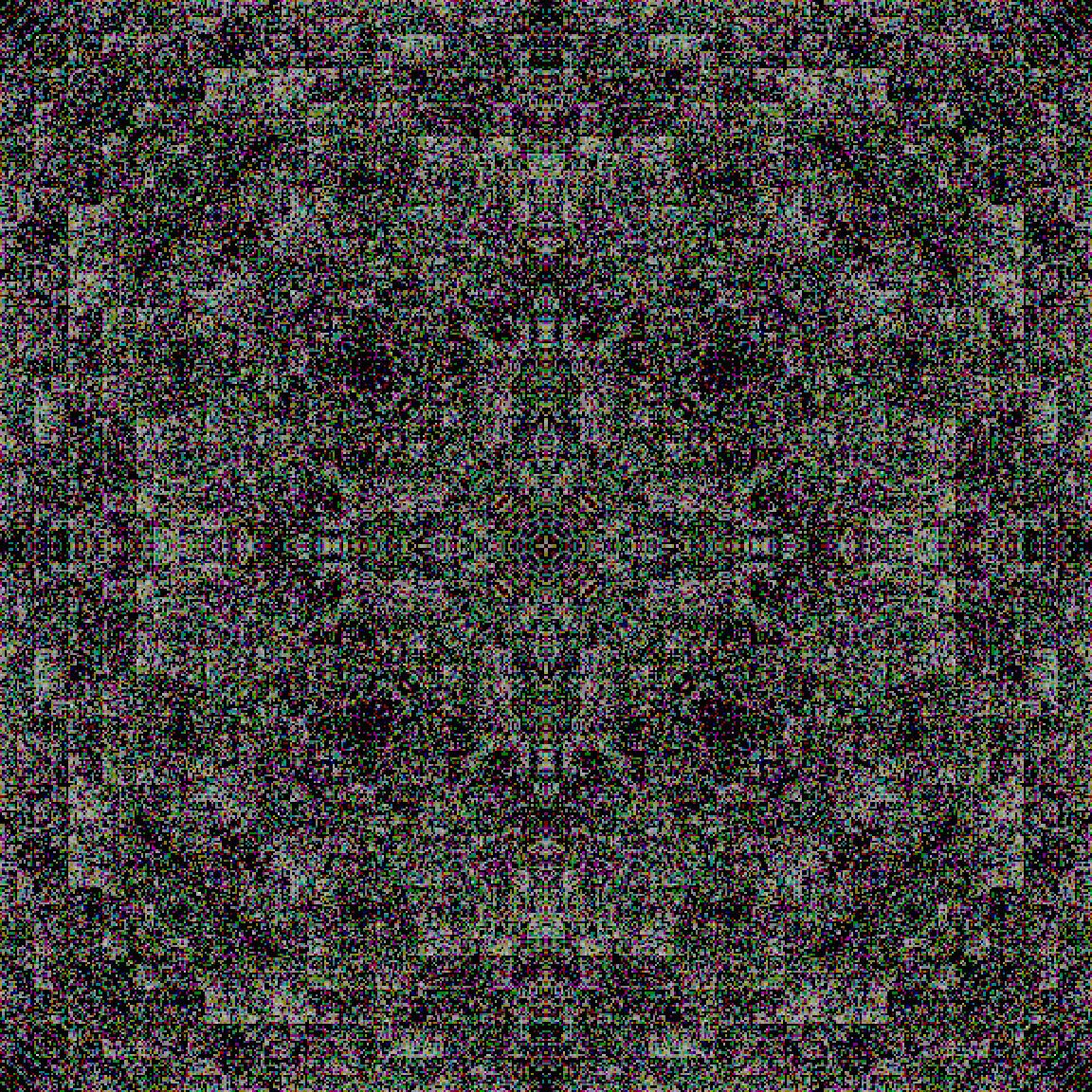Possible Issues

By default, TextToKaleidoscope will produce very dark images if given a short text:

 In:=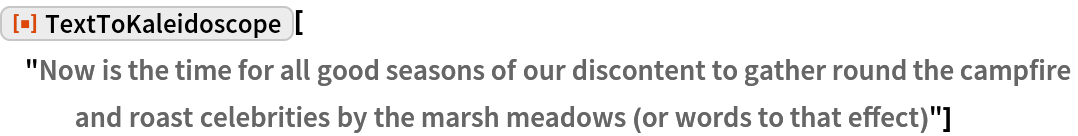Out=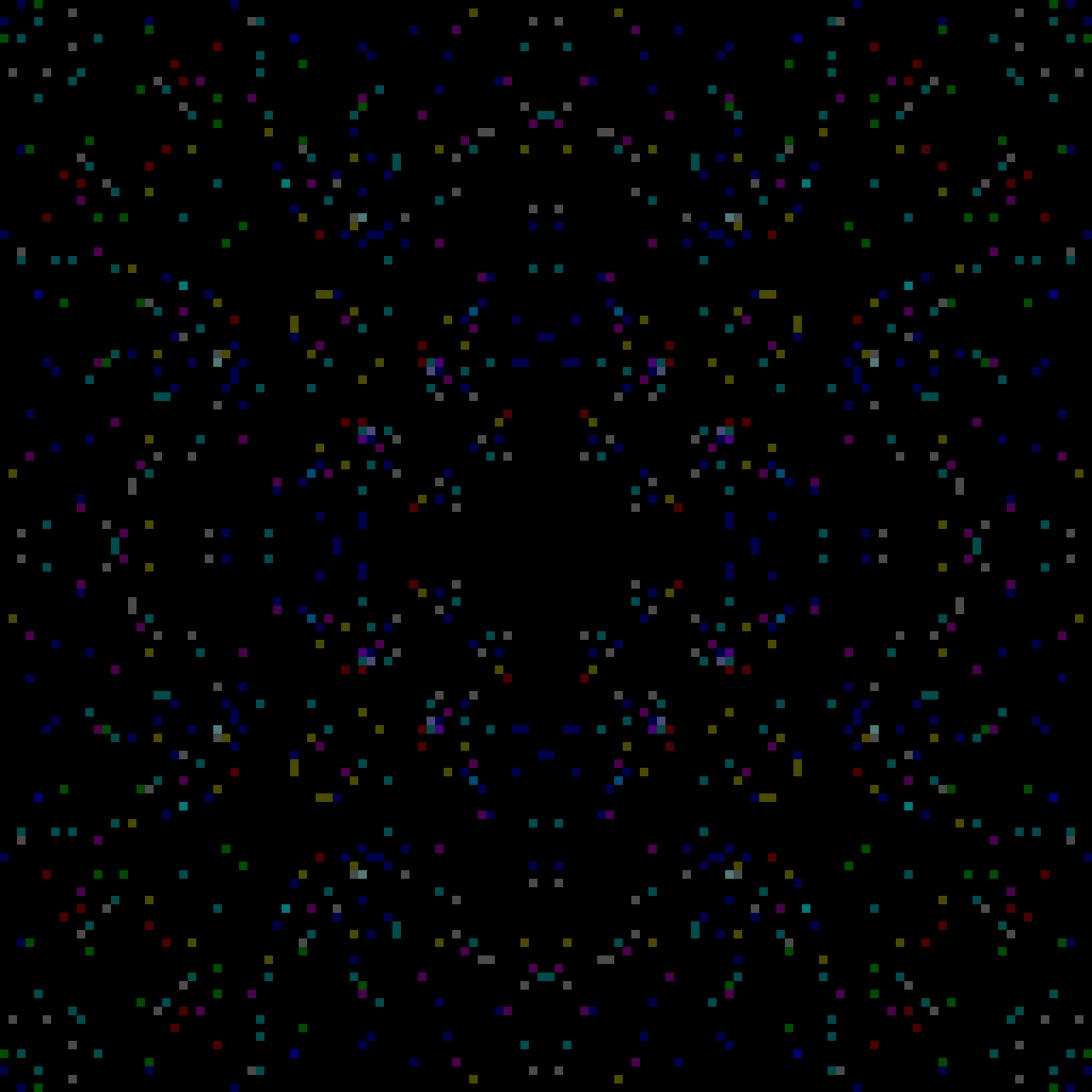Using a lower pixelation value for short text can produce brighter images:

 In:=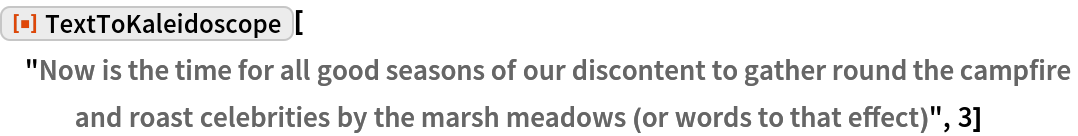Out=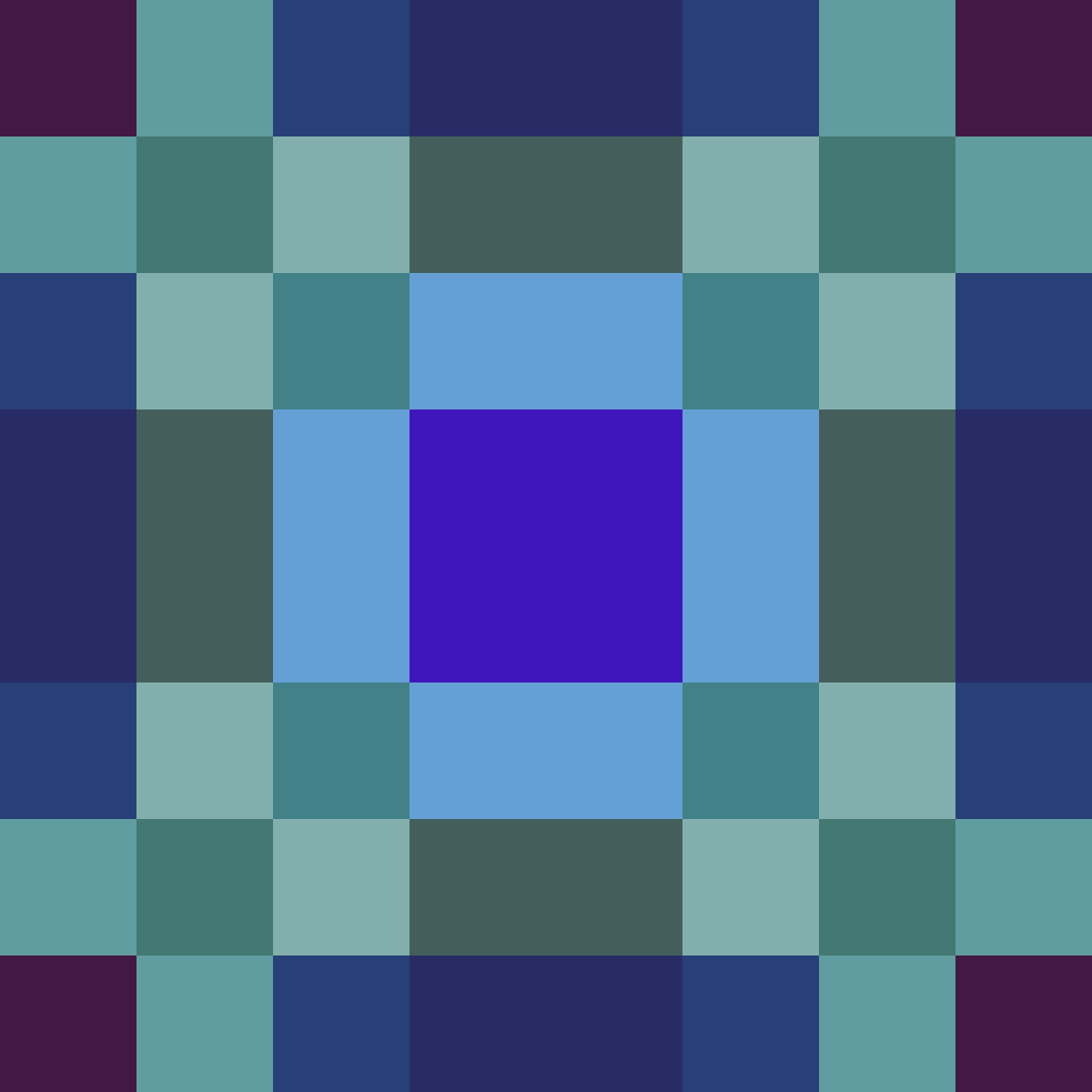Images can be lightened explicitly using Lighter:

 In:=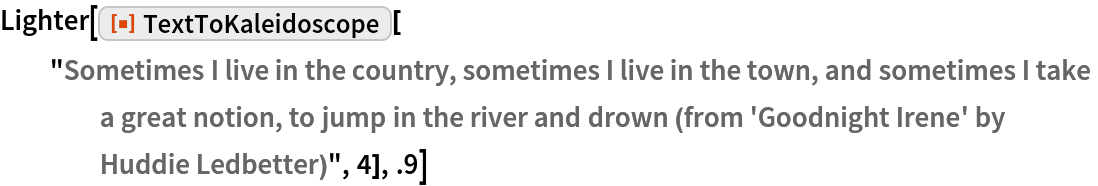Out=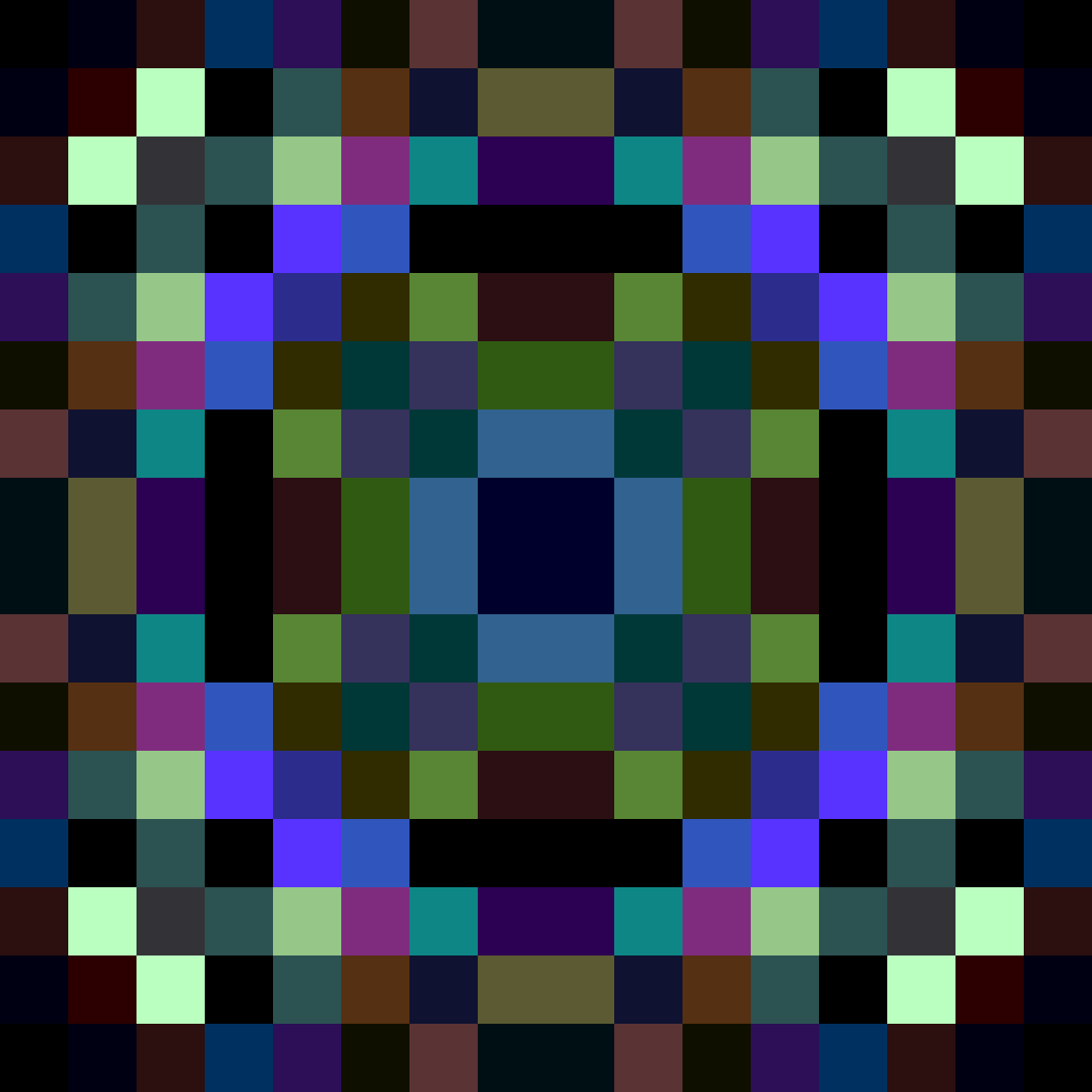Requirements

Wolfram Language 11.3 (March 2018) or above